Anúncio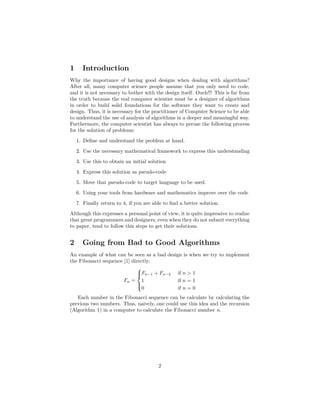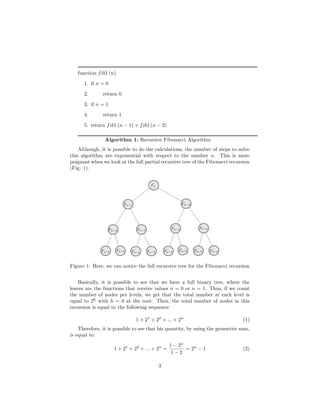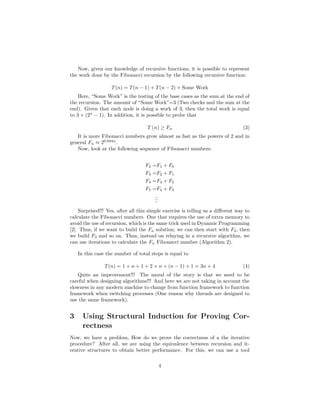Anúncio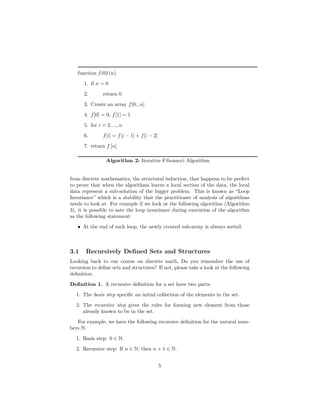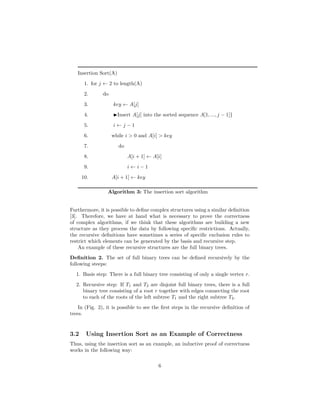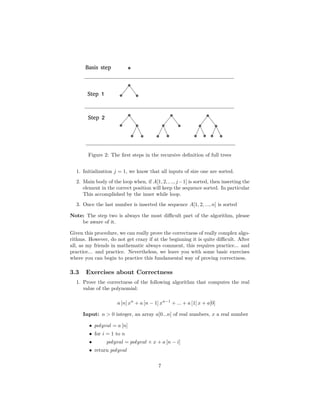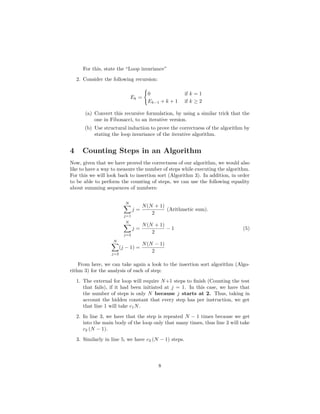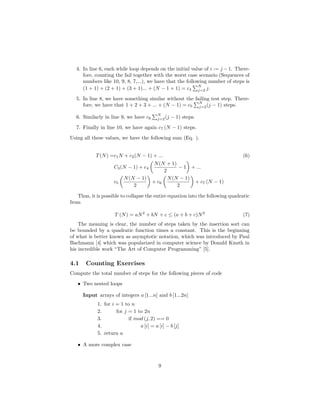Anúncio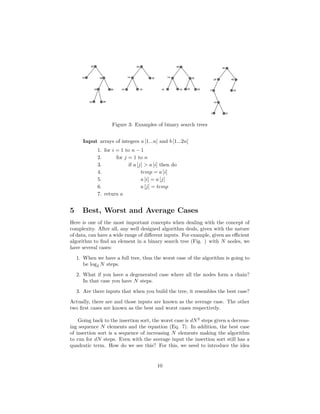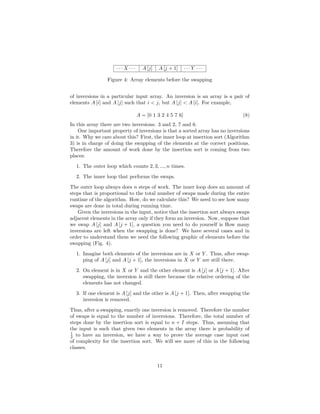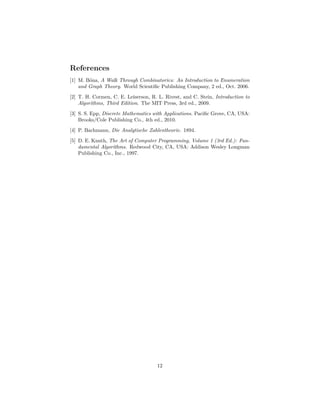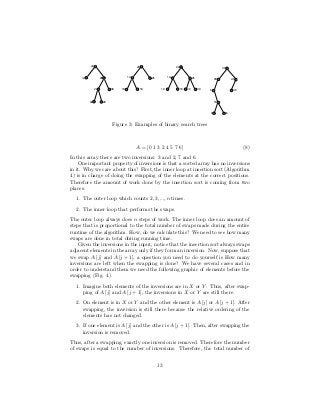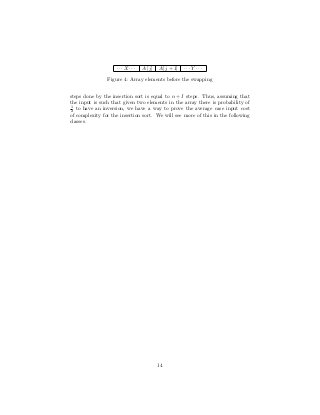Anúncio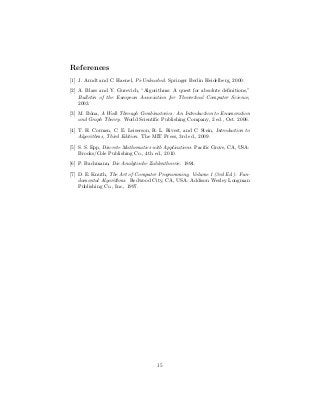Próximos SlideShares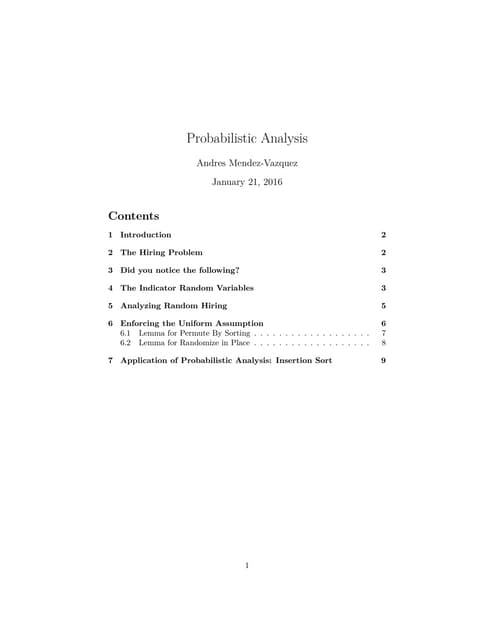03 notes
Carregando em ... 3
1 de 15
Anúncio

### 01 Notes Introduction Analysis of Algorithms Notes

1. Introduction Andres Mendez-Vazquez September 6, 2015 To my dear Fabi... For her strength and understanding in those rainy days... 1
2. Contents 1 Introduction 3 2 The absolute deﬁnition of an algorithm 3 3 Going from Bad to Good Algorithms 5 4 Using Structural Induction for Proving Correctness 7 4.1 Recursively Deﬁned Sets and Structures . . . . . . . . . . . . . . 7 4.2 Using Insertion Sort as an Example of Correctness . . . . . . . . 9 4.3 Exercises about Correctness . . . . . . . . . . . . . . . . . . . . . 10 5 Counting Steps in an Algorithm 10 5.1 Counting Exercises . . . . . . . . . . . . . . . . . . . . . . . . . . 11 6 Best, Worst and Average Cases 12 References 15 2
3. 1 Introduction Why the importance of having good designs when dealing with algorithms? After all, many computer science people assume that you only need to code, and it is not necessary to bother with the design itself. Ouch!!! This is far from the truth because the real computer scientist must be a designer of algorithms in order to build solid foundations for the software they want to create and design. Thus, it is necessary for the practitioner of Computer Science to be able to understand the use of analysis of algorithms in a deeper and meaningful way. Furthermore, the computer scientist has always to peruse the following process for the solution of problems: 1. Deﬁne and understand the problem at hand. 2. Use the necessary mathematical framework to express this understanding 3. Use this to obtain an initial solution 4. Express this solution as pseudo-code 5. Move that pseudo-code to target language to be used. 6. Using your tools from hardware and mathematics improve over the code 7. Finally return to 4, if you are able to ﬁnd a better solution. Although this expresses a personal point of view, it is quite impressive to realize that great programmers and designers, even when they do not submit everything to paper, tend to follow this steps to get their solutions. 2 The absolute deﬁnition of an algorithm Although the history of algorithms is as old as Archimedes approximation of π , there is still a lack of an absolute deﬁnition of an algorithm when you take in account concepts as diﬀerent as: 1. Parallel Algorithms. 2. Distributed Algorithms. 3. Quantum Algorithms. 4. Approximation Algorithms. 5. Heuristics. Thus, the quest for the absolute deﬁnition is still going on  in the hopes that one day, we will be able to deﬁne what is an algorithm. Nevertheless, manly because we concrete, we will use for this course the deﬁnition by Kolmogorov: 3
4. Deﬁnition 1. An algorithmic process has the following properties: 1. An algorithmic process splits into steps whose complexity is bounded in advance • i.e., the bound is independent of the input and the current state of the computation. 2. Each step consists of a direct and immediate transformation of the current state. 3. This transformation applies only to the active part of the state and does not alter the remainder of the state. 4. The size of the active part is bounded in advance. 5. The process runs until either the next step is impossible or a signal says the solution has been reached. For example, while looking at the pseudo-code (Algorithm 1), we are able to notice the following: • The state of the algorithm is maintainer by the variables A and i. • The active part is always an element A [i] and i. In addition, the trans- formation only applies to those parts. • Clearly each step is bounded by the time of executing the instructions inside the loop body. • Finally, the process continues until i becomes 0. Given the previous example, this deﬁnition is actually a really complete one allowing us to obtain a deeper understanding of what an algorithm is or can be. function Bounded (n, A [1...n]) 1. i = n 2. while i > 0 3. A [i] = n − i 4. i = i − 1 5. return A Algorithm 1: Example for the Kolmogorov deﬁnition 4
5. 3 Going from Bad to Good Algorithms An example of what can be seen as a bad design is when we try to implement the Fibonacci sequence  directly: Fn =    Fn−1 + Fn−2 if n > 1 1 if n = 1 0 if n = 0 Each number in the Fibonacci sequence can be calculate by calculating the previous two numbers. Thus, naively, one could use this idea and the recursion (Algorithm 2) in a computer to calculate the Fibonacci number n. function fib1 (n) 1. if n = 0 2. return 0 3. if n = 1 4. return 1 5. return fib1 (n − 1) + fib1 (n − 2) Algorithm 2: Recursive Fibonacci Algorithm Although, it is possible to do the calculations, the number of steps to solve this algorithm are exponential with respect to the number n. This is more poignant when we look at the full partial recursive tree of the Fibonacci recursion (Fig. 1). Figure 1: Here, we can notice the full recursive tree for the Fibonacci recursion 5
6. Basically, it is possible to see that we have a full binary tree, where the leaves are the functions that receive values n = 0 or n = 1. Thus, if we count the number of nodes per levels, we get that the total number at each level is equal to 2h with h = 0 at the root. Then, the total number of nodes in this recursion is equal to the following sequence: 1 + 21 + 22 + ... + 2n (1) Therefore, it is possible to see that his quantity, by using the geometric sum, is equal to: 1 + 21 + 22 + ... + 2n = 1 − 2n 1 − 2 = 2n − 1 (2) Now, given our knowledge of recursive functions, it is possible to represent the work done by the Fibonacci recursion by the following recursive function: T(n) = T(n − 1) + T(n − 2) + Some Work Here, “Some Work” is the testing of the base cases as the sum at the end of the recursion. The amount of “Some Work”=3 (Two checks and the sum at the end). Given that each node is doing a work of 3, then the total work is equal to 3 × (2n − 1). In addition, it is possible to probe that T (n) ≥ Fn (3) It is more Fibonacci numbers grow almost as fast as the powers of 2 and in general Fn ≈ 20.694n . Now, look at the following sequence of Fibonacci numbers: F2 =F1 + F0 F3 =F2 + F1 F4 =F3 + F2 F5 =F4 + F3 ... ... Surprised!!! Yes, after all this simple exercise is telling us a diﬀerent way to calculate the Fibonacci numbers. One that requires the use of extra memory to avoid the use of recursion, which is the same trick used in Dynamic Programming . Thus, if we want to build the Fn solution, we can then start with F2, then we build F3 and so on. Thus, instead on relaying in a recursive algorithm, we can use iterations to calculate the Fn Fibonacci number (Algorithm 3). In this case the number of total steps is equal to T(n) = 1 + n + 1 + 2 + n + (n − 1) + 1 = 3n + 4 (4) 6
7. function fib2 (n) 1. if n = 0 2. return 0 3. Create an array f[0...n] 4. f = 0, f = 1 5. for i = 2, ..., n 6. f[i] = f[i − 1] + f[i − 2] 7. return f [n] Algorithm 3: Iterative Fibonacci Algorithm Quite an improvement!!! The moral of the story is that we need to be careful when designing algorithms!!! And here we are not taking in account the slowness in any modern machine to change from function framework to function framework when switching processes (One reason why threads are designed to use the same framework). 4 Using Structural Induction for Proving Cor- rectness Now, we have a problem, How do we prove the correctness of a the iterative procedure? After all, we are using the equivalence between recursion and it- erative structures to obtain better performance. For this, we can use a tool from discrete mathematics, the structural induction, that happens to be perfect to prove that when the algorithms leaves a local section of the data, the local data represent a sub-solution of the bigger problem. This is known as “Loop Invariance” which is a stability that the practitioner of analysis of algorithms needs to look at. For example if we look at the following algorithm (Algorithm 4), it is possible to sate the loop invariance during execution of the algorithm as the following statement: • At the end of each loop, the newly created sub-array is always sorted. 4.1 Recursively Deﬁned Sets and Structures Looking back to our course on discrete math, Do you remember the use of recursion to deﬁne sets and structures? If not, please take a look at the following deﬁnition: 7
8. Insertion Sort(A) 1. for j ← 2 to length(A) 2. do 3. key ← A[j] 4. Insert A[j] into the sorted sequence A[1, ..., j − 1]} 5. i ← j − 1 6. while i > 0 and A[i] > key 7. do 8. A[i + 1] ← A[i] 9. i ← i − 1 10. A[i + 1] ← key Algorithm 4: The insertion sort algorithm Deﬁnition 2. A recursive deﬁnition for a set have two parts: 1. The basis step speciﬁc an initial collection of the elements in the set. 2. The recursive step gives the rules for forming new element from those already known to be in the set. For example, we have the following recursive deﬁnition for the natural num- bers N. 1. Basis step: 0 ∈ N. 2. Recursive step: If n ∈ N, then n + 1 ∈ N. Furthermore, it is possible to deﬁne complex structures using a similar deﬁnition . Therefore, we have at hand what is necessary to prove the correctness of complex algorithms, if we think that these algorithms are building a new structure as they process the data by following speciﬁc restrictions. Actually, the recursive deﬁnitions have sometimes a series of speciﬁc exclusion rules to restrict which elements can be generated by the basis and recursive step. An example of these recursive structures are the full binary trees. Deﬁnition 3. The set of full binary trees can be deﬁned recursively by the following steeps: 1. Basis step: There is a full binary tree consisting of only a single vertex r. 8
9. Basis step Step 1 Step 2 Figure 2: The ﬁrst steps in the recursive deﬁnition of full trees 2. Recursive step: If T1 and T2 are disjoint full binary trees, there is a full binary tree consisting of a root r together with edges connecting the root to each of the roots of the left subtree T1 and the right subtree T2. In (Fig. 2), it is possible to see the ﬁrst steps in the recursive deﬁnition of trees. 4.2 Using Insertion Sort as an Example of Correctness Thus, using the insertion sort as an example, an inductive proof of correctness works in the following way: 1. Initialization j = 1, we know that all inputs of size one are sorted. 2. Main body of the loop when, if A[1, 2, , .., j−1] is sorted, then inserting the element in the correct position will keep the sequence sorted. In particular This accomplished by the inner while loop. 3. Once the last number is inserted the sequence A[1, 2, ..., n] is sorted Note: The step two is always the most diﬃcult part of the algorithm, please be aware of it. Given this procedure, we can really prove the correctness of really complex algo- rithms. However, do not get crazy if at the beginning it is quite diﬃcult. After all, as my friends in mathematic always comment, this requires practice... and practice... and practice. Nevertheless, we leave you with some basic exercises where you can begin to practice this fundamental way of proving correctness. 9
10. 4.3 Exercises about Correctness 1. Prove the correctness of the following algorithm that computes the real value of the polynomial: a [n] xn + a [n − 1] xn−1 + ... + a  x + a Input: n > 0 integer, an array a[0...n] of real numbers, x a real number • polyval = a [n] • for i = 1 to n • polyval = polyval × x + a [n − i] • return polyval For this, state the “Loop invariance” 2. Consider the following recursion: Ek = 0 if k = 1 Ek−1 + k + 1 if k ≥ 2 (a) Convert this recursive formulation, by using a similar trick that the one in Fibonacci, to an iterative version. (b) Use structural induction to prove the correctness of the algorithm by stating the loop invariance of the iterative algorithm. 5 Counting Steps in an Algorithm Now, given that we have proved the correctness of our algorithm, we would also like to have a way to measure the number of steps while executing the algorithm. For this we will look back to insertion sort (Algorithm 4). In addition, in order to be able to perform the counting of steps, we can use the following equalities about sequences of numbers (Eq. 5). N j=1 j = N(N + 1) 2 (Arithmetic sum). N j=2 j = N(N + 1) 2 − 1 (5) N j=2 (j − 1) = N(N − 1) 2 From here, we can take again a look to the insertion sort algorithm (Algo- rithm 4) for the analysis of each of step: 10
11. 1. The external for loop will require N+1 steps to ﬁnish (Counting the test that fails), if it had been initiated at j = 1. In this case, we have that the number of steps is only N because j starts at 2. Thus, taking in account the hidden constant that every step has per instruction, we get that line 1 will take c1N. 2. In line 3, we have that the step is repeated N − 1 times because we get into the main body of the loop only that many times, thus line 3 will take c2 (N − 1). 3. Similarly in line 5, we have c3 (N − 1) steps. 4. In line 6, each while loop depends on the initial value of i := j −1. There- fore, counting the fail together with the worst case scenario (Sequences of numbers like 10, 9, 8, 7,...), we have that the following number of steps is (1 + 1) + (2 + 1) + (3 + 1)... + (N − 1 + 1) = c4 N j=2 j. 5. In line 8, we have something similar without the failing test step. There- fore, we have that 1 + 2 + 3 + ... + (N − 1) = c5 N j=2(j − 1) steps. 6. Similarly in line 9, we have c6 N j=2(j − 1) steps. 7. Finally in line 10, we have again c7 (N − 1) steps. Using all these values, we have the following sum (Eq. ). T(N) =c1N + c2(N − 1) + ... (6) C3(N − 1) + c4 N(N + 1) 2 − 1 + ... c5 N(N − 1) 2 + c6 N(N − 1) 2 + c7 (N − 1) Thus, it is possible to collapse the entire equation into the following quadratic from T (N) = aN2 + bN + c ≤ (a + b + c)N2 (7) The meaning is clear, the number of steps taken by the insertion sort can be bounded by a quadratic function times a constant. This is the beginning of what is better known as asymptotic notation, which was introduced by Paul Bachmann  which was popularized in computer science by Donald Knuth in his incredible work “The Art of Computer Programming” . 5.1 Counting Exercises Compute the total number of steps for the following pieces of code • Two nested loops 11
12. Input arrays of integers a [1...n] and b [1...2n] 1. for i = 1 to n 2. for j = 1 to 2n 3. if mod (j, 2) == 0 4. a [i] = a [i] − b [j] 5. return a • A more complex case Input arrays of integers a [1...n] and b [1...2n] 1. for i = 1 to n − 1 2. for j = 1 to n 3. if a [j] > a [i] then do 4. temp = a [i] 5. a [i] = a [j] 6. a [j] = temp 7. return a 6 Best, Worst and Average Cases Here is one of the most important concepts when dealing with the concept of complexity. After all, any well designed algorithm deals, given with the nature of data, can have a wide range of diﬀerent inputs. For example, given an eﬃcient algorithm to ﬁnd an element in a binary search tree (Fig. 3) with N nodes, we have several cases: 1. When we have a full tree, thus the worst case of the algorithm is going to be log2 N steps. 2. What if you have a degenerated case where all the nodes form a chain? In that case you have N steps. 3. Are there inputs that when you build the tree, it resembles the best case? Actually, there are and those inputs are known as the average case. The other two ﬁrst cases are known as the best and worst cases respectively. Going back to the insertion sort, the worst case is dN2 steps given a decreas- ing sequence N elements and the equation (Eq. 7). In addition, the best case of insertion sort is a sequence of increasing N elements making the algorithm to run for dN steps. Even with the average input the insertion sort still has a quadratic term. How do we see this? For this, we need to introduce the idea of inversions in a particular input array. An inversion is an array is a pair of elements A [i] and A [j] such that i < j, but A [j] < A [i]. For example, 12
13. 20 14 28 24 22 26 30 20 14 10 18 25 20 14 10 18 25 22 30 30 20 5 10 7 15 45 35 Figure 3: Examples of binary search trees A = [0 1 3 2 4 5 7 6] (8) In this array there are two inversions: 3 and 2, 7 and 6. One important property of inversions is that a sorted array has no inversions in it. Why we care about this? First, the inner loop at insertion sort (Algorithm 4) is in charge of doing the swapping of the elements at the correct positions. Therefore the amount of work done by the insertion sort is coming from two places: 1. The outer loop which counts 2, 3, ..., n times. 2. The inner loop that performs the swaps. The outer loop always does n steps of work. The inner loop does an amount of steps that is proportional to the total number of swaps made during the entire runtime of the algorithm. How, do we calculate this? We need to see how many swaps are done in total during running time. Given the inversions in the input, notice that the insertion sort always swaps adjacent elements in the array only if they form an inversion. Now, suppose that we swap A [j] and A [j + 1], a question you need to do yourself is How many inversions are left when the swapping is done? We have several cases and in order to understand them we need the following graphic of elements before the swapping (Fig. 4). 1. Imagine both elements of the inversions are in X or Y . Thus, after swap- ping of A [j] and A [j + 1], the inversions in X or Y are still there. 2. On element is in X or Y and the other element is A [j] or A [j + 1]. After swapping, the inversion is still there because the relative ordering of the elements has not changed. 3. If one element is A [j] and the other is A [j + 1]. Then, after swapping the inversion is removed. Thus, after a swapping, exactly one inversion is removed. Therefore the number of swaps is equal to the number of inversions. Therefore, the total number of 13
14. · · · X · · · A [j] A [j + 1] · · · Y · · · Figure 4: Array elements before the swapping steps done by the insertion sort is equal to n + I steps. Thus, assuming that the input is such that given two elements in the array there is probability of 1 2 to have an inversion, we have a way to prove the average case input cost of complexity for the insertion sort. We will see more of this in the following classes. 14
15. References  J. Arndt and C. Haenel, Pi-Unleashed. Springer Berlin Heidelberg, 2000.  A. Blass and Y. Gurevich, “Algorithms: A quest for absolute deﬁnitions,” Bulletin of the European Association for Theoretical Computer Science, 2003.  M. Bóna, A Walk Through Combinatorics: An Introduction to Enumeration and Graph Theory. World Scientiﬁc Publishing Company, 2 ed., Oct. 2006.  T. H. Cormen, C. E. Leiserson, R. L. Rivest, and C. Stein, Introduction to Algorithms, Third Edition. The MIT Press, 3rd ed., 2009.  S. S. Epp, Discrete Mathematics with Applications. Paciﬁc Grove, CA, USA: Brooks/Cole Publishing Co., 4th ed., 2010.  P. Bachmann, Die Analytische Zahlentheorie. 1894.  D. E. Knuth, The Art of Computer Programming, Volume 1 (3rd Ed.): Fun- damental Algorithms. Redwood City, CA, USA: Addison Wesley Longman Publishing Co., Inc., 1997. 15
Anúncio